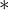# Optimal alignment – Needleman Wunsch Algorithm

Needleman Wunsch Algorithm utilizes Dynamic programming to solve a traditional problem of bioinformatics: align 2 sequences of nucleotide in the optimal way such that the number of matched nucleotides is maximized and the number of deletions and insertions is minimized.

For example, we have 2 nucleotide sequences like below:

The alignment could be:

For clarification: the stricken-through ones are the ones to be deleted. In the above alignment, the first sequence is reduced to 4 nucleotides (A at position 1 and C at position 5 are deleted), the second sequence is also reduced to 4 nucleotides (the last 2 nucleotides are deleted).

After deletions, the 2 sequences become:

In the above alignment, we delete nucleotide 4 times and get matching of length 4. Hence, we have the aligning valuation equals (-4)delete_cost + 4match_reward.

Needleman-Wunsch Algorithm

As we stated at the beginning of the blog, the Needleman-Wunsch Algorithm solves this optimal alignment problem by using dynamic programming.

From now, we will call the 2 sequences: s1 and s2.

we define a 2-D array dp with size n x m, while n is the length of s1 and m is the length of s2.
dp[i][j] represents the maximum aligning valuation we can have when aligning the first i nucleotide of sequence 1 with the first j nucleotide of sequence 2.

Initially, we set:

```for (int i = 0; i <= n; ++i)
dp[i] = - delete_cost * i;
for (int i = 0; i <= m; ++i)
dp[i] = - delete_cost * i;```

Then, for each pair of i and j, we have:

```dp[i][j] = max(dp[i][j-1] - delete_cost,  dp[i-1][j] - delete_cost);
if (s1[i] == s2[j])
dp[i][j] = max(dp[i][j], dp[i-1][j-1] + match_reward);```

The result (optimal aligning valuation) is stored in dp[n][m].

Implementation

Unfortunately, I don’t find any reliable libraries in python that support this algorithm. Hence, we will have to write our own.

As for the optimization of speed, we write this on C++.

The sample code is below. Depends on different situations, we may tweak the code a little bit to fit our cases.

```// Sample usage :
//	vector<string> s1 = {"One", "Two", "There"};
//	vector<string> s2 = {"One", "Two", "Four"};
//	NeedlemanWunsch needle(0, 1);
//	cout << needle.computeMatchValue(s1, s2) << endl;
struct NeedlemanWunsch {
vector< vector<int> > dp;
int delete_cost;
int match_reward;

NeedlemanWunsch(int delete_cost_=0, int match_reward_=1) {
delete_cost = delete_cost_;
match_reward = match_reward_;
}

float computeMatchValue(vector<string> s1, vector<string> s2) {
// get size of each vector
int n = s1.size();
int m = s2.size();
// prepare a dp matrix
dp.resize(n+1, vector<int>(m+1, 0));
// initialize dp matrix
for (int i = 0; i <= n; ++i)
dp[i] = -delete_cost*i;
for (int j = 0; j <= m; ++j)
dp[j] = -delete_cost*j;
// compute dp
for (int i = 1; i <= n; ++i)
for (int j = 1; j <= m; ++j) {
dp[i][j] = max(dp[i-1][j] - delete_cost,
dp[i][j-1] - delete_cost);
if (s1[i-1] == s2[j-1])
dp[i][j] = max(dp[i][j],
dp[i-1][j-1] + match_reward);
}

return dp[n][m];

}

};```

Apart from aligning nucleotide, we can use this algorithm to compute alignments of words (consider each character to be a nucleotide) or alignment of sentences (consider each word to be a nucleotide).

References:

• Wikipedia’s page on Needleman-Wunsch algorithm: link